Custom SearchSUMMARY This chapter has presented information on the characteristics of transmission lines. The information that follows summarizes the important points of this chapter. TRANSMISSION LINES are devices for guiding electrical energy from one point to another. INPUT IMPEDANCE is the ratio of voltage to current at the input end of a transmission line. OUTPUT IMPEDANCE is the ratio of voltage to current at the output end of the line. TWO-WIRE OPEN LINES are parallel lines and have uses such as power lines, rural telephone lines, and telegraph lines. This type of line has high radiation losses and is subject to noise pickup.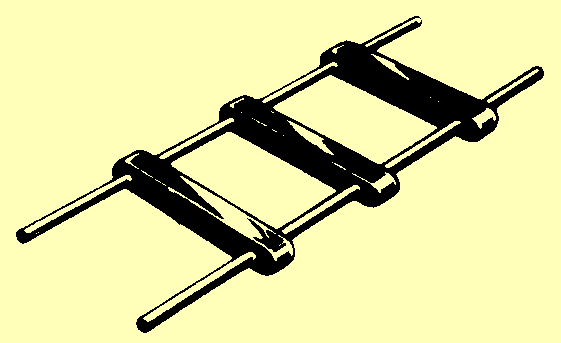TWIN LEAD has parallel lines and is most often used to connect televisions to their antennas.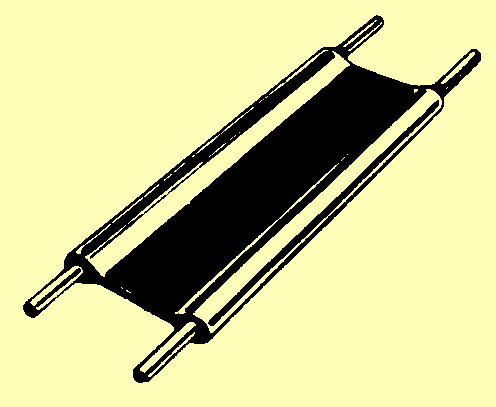A TWISTED PAIR consists of two insulated wires twisted together. This line has high insulation loss.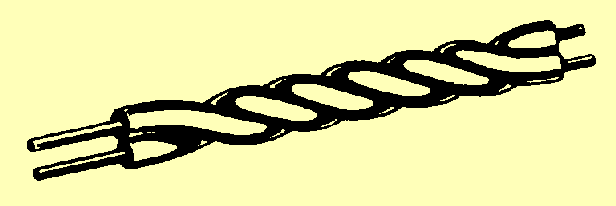A SHIELDED PAIR has parallel conductors separated by a solid dielectric and surrounded by copper braided tubing. The conductors are balanced to ground.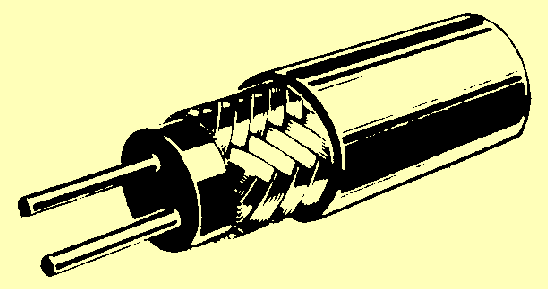RIGID COAXIAL LINE contains two concentric conductors insulated from each other by spacers. Some rigid coaxial lines are pressurized with an inert gas to prevent moisture from entering. High-frequency losses are less than with other lines.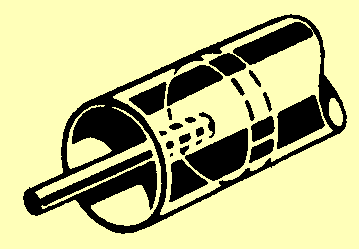FLEXIBLE COAXIAL LINES consist of a flexible inner conductor and a concentric outer conductor of metal braid. The two are separated by a continuous insulating material.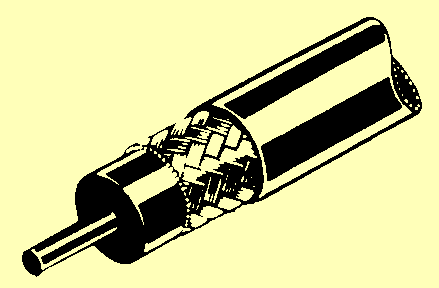WAVEGUIDES are hollow metal tubes used to transfer energy from one point to another. The energy travels slower in a waveguide than in free space.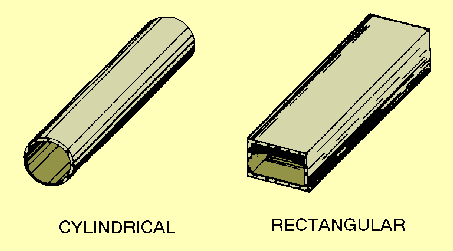COPPER LOSSES can result from power (I2R) loss, in the form of heat, or skin effect. These losses decrease the conductivity of a line. DIELECTRIC LOSSES are caused by the heating of the dielectric material between conductors, taking power from the source. RADIATION AND INDUCTION LOSSES are caused by part of the electromagnetic fields of a conductor being dissipated into space or nearby objects. A transmission line is either electrically LONG or SHORT if its physical length is not equal to 1/4l for the frequency it is to carry. LUMPED CONSTANTS are theoretical properties (inductance, resistance, and capacitance) of a transmission line that are lumped into a single component.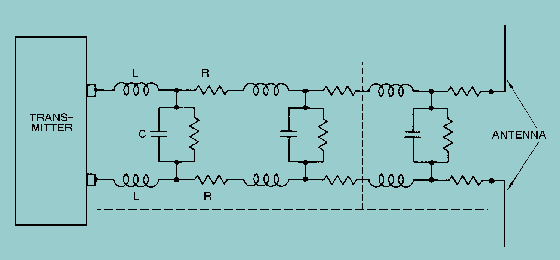DISTRIBUTED CONSTANTS are constants of inductance, capacitance and resistance that are distributed along the transmission line.LEAKAGE CURRENT flows between the wires of a transmission line through the dielectric. The dielectric acts as a resistor.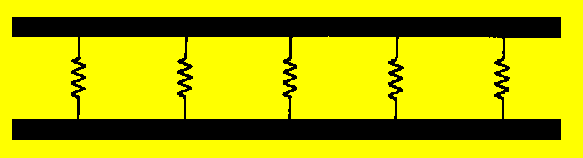An ELECTROMAGNETIC FIELD exists along transmission line when current flows through it.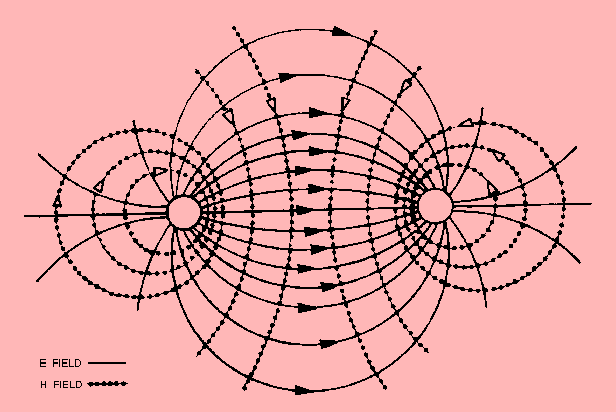CHARACTERISTIC IMPEDANCE, Z0, is the ratio of E to I at every point along the line. For maximum transfer of electrical power, the characteristic impedance and load impedance must be matched.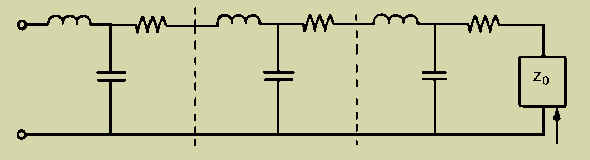The VELOCITY at which a wave travels over a given length of transmission line can be found by using the formula: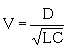A transmission line that is not terminated in its characteristic impedance is said to be FINITE. When dc is applied to an OPEN-ENDED line, the voltage is reflected back from the open end without any change in polarity, amplitude, or shape. Current is reflected back with the same amplitude and shape but with opposite polarity. When dc is applied to a SHORT-CIRCUITED line, the current is reflected back with the same amplitude, and polarity. The voltage is reflected back with the same amplitude but with opposite polarity. When ac is applied to an OPEN-END line, voltage is always reflected back in phase with the incident wave and current is reflected back out of phase.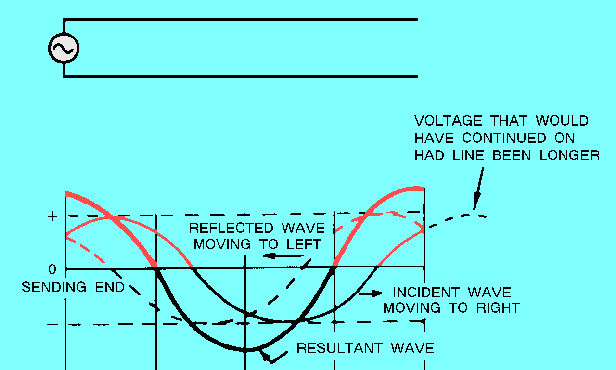When ac is applied to a SHORT-CIRCUITED line, voltage is reflected in opposite phase, while current is reflected in phase.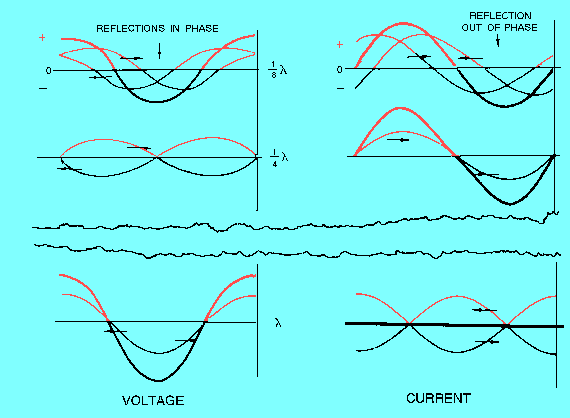A NONRESONANT line has NO STANDING WAVES of current and voltage and is either infinitely long or terminated in its characteristic impedance. A RESONANT line has STANDING WAVES of current and voltage and is of finite length and is NOT terminated in its characteristic impedance. On an open-ended resonant line, and at all odd 1/4 l points, the voltage is minimum, the current is maximum, and the impedance is minimum. At all even 1/4 l points, the voltage is maximum, the current is minimum and the impedance is maximum.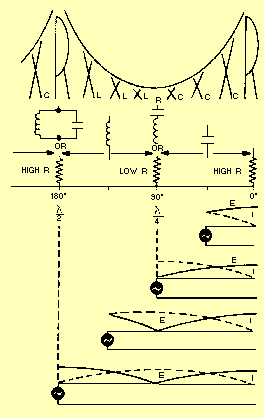There are a variety of TERMINATIONS for rf lines. Each termination has an effect on the standing waves on the line.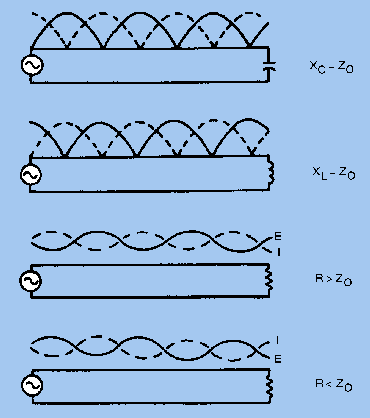A transmission line can be terminated in its characteristic impedance as an open- or short-circuit, or in capacitance or inductance. Whenever the termination on a transmission line is NOT EQUAL TO Z0, there are reflections on the line. The amount of voltage reflected may be found by using the equation: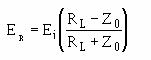When the termination on a transmission line EQUALS Z0, there is NO reflected voltage. The measurement of standing waves on a transmission line yields information about operating conditions. If there are NO standing waves, the termination for that line is correct and maximum power transfer takes place. The STANDING WAVE RATIO is the measurement of maximum voltage (current) to minimum voltage (current) on a transmission line and measures the perfection of the termination of the line. A ratio of 1:1 describes a line terminated in its characteristic impedance.Integrated Publishing, Inc. - A (SDVOSB) Service Disabled Veteran Owned Small Business